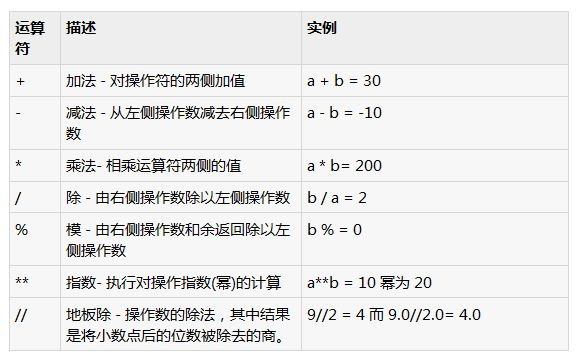# 举例讲解python中的算数运算符的用法#!/usr/bin/python

a = 21
b = 10
c = 0

c = a + b
print “line 1 – value of c is “, c

c = a – b
print “line 2 – value of c is “, c

c = a * b
print “line 3 – value of c is “, c

c = a / b
print “line 4 – value of c is “, c

c = a % b
print “line 5 – value of c is “, c

a = 2
b = 3
c = a**b
print “line 6 – value of c is “, c

a = 10
b = 5
c = a//b
print “line 7 – value of c is “, c

line 1 – value of c is 31
line 2 – value of c is 11
line 3 – value of c is 210
line 4 – value of c is 2
line 5 – value of c is 1
line 6 – value of c is 8
line 7 – value of c is 2